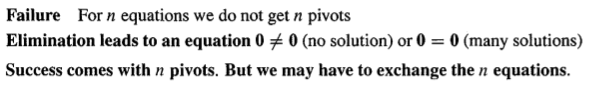Abstract: 从小学解方程的消元开始，推导出线性代数的知识，包括先关矩阵计算
Keywords: Eliminate 消元，Pivot 主元，Row Exchange 行交换，Upper Triangular 上三角矩阵

# 消元

$$x+y=1\\ x-y=0\\$$

$$-x-y=-1……..(1_{temp})$$

$$-2y=-1………(2)$$

$$x+y=1\\ 0x+y=\frac{1}{2}$$

## 上三角矩阵(Upper Triangular Matrix)

$$\left(\begin{array}{cccc} a_{11} & a_{12} & \cdots & a_{1n} \\ &a_{22} & \cdots &a_{2n} \\ & & \ddots & \vdots \\ & & &a_{nn} \end{array}\right)$$

$$U\textbf{x}=\textbf{c}$$

## 主值(Pivot)

eg：

$$l_{ij}=\frac{entry\,to\,eliminate\,in\,row\,i}{pivot\,in\,row\,j}$$
Pivot in Row j 就是当前的主元

## 消元失败(Eliminate Faile)0%